NCERT Solutions for Class 7 Maths Chapter 8 Comparing Quantities Ex 8.3 are part of NCERT Solutions for Class 7 Maths. Here we have given NCERT Solutions for Class 7 Maths Chapter 8 Comparing Quantities Ex 8.3.

 Board CBSE Textbook NCERT Class Class 7 Subject Maths Chapter Chapter 8 Chapter Name Comparing Quantities Exercise Ex 8.3 Number of Questions Solved 11 Category NCERT Solutions

## NCERT Solutions for Class 7 Maths Chapter 8 Comparing Quantities Ex 8.3

Question 1.
Tell what is the profit or loss in the following transactions. Also find profit percent or loss per cent in each case.

(a) Gardening shears bought for ₹ 250 and sold for ₹ 325.
(b) A refrigerater bought for ₹ 12,000 and sold at ₹ 13,500.
(c) Acupboard bought for ₹ 2,500 and sold at ₹ 3,000.
(d) A skirt bought for ₹ 250 and sold at ₹ 150.

Solution: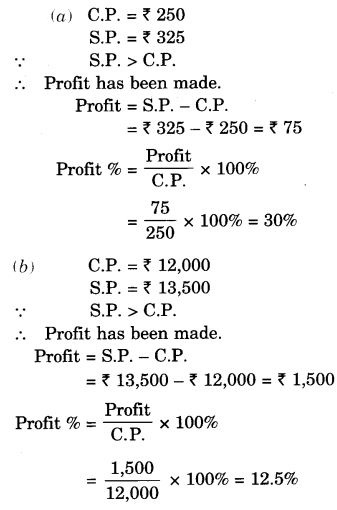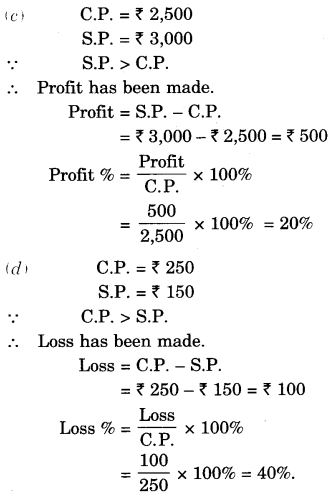Question 2.
Convert each part of the ratio to percentage

(a) 3 : 1
(b) 2 : 3 : 5
(c) 1 : 4
(d) 1 : 2 : 5

Solution: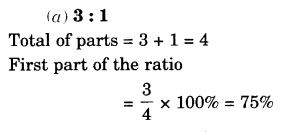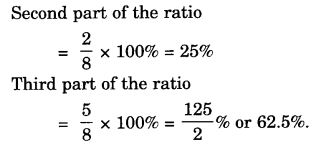Question 3.
The population of a city decreased from 25,000 to 24,500. Find the percentage decrease.
Solution:Question 4.
Arun bought a car for ₹ 3,50,000. The next year, the price went upto ₹ 3,70,000. What was the percentage of the price increase?
Solution: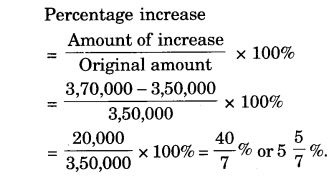Question 5.
1 buy a T.V. for ₹ 10,000 and sell it at a profit of 20%. How much money do I get for it?
Solution:
C.P. = ₹ 10,000
Profit = 20% of C.P.
= 20% of ₹ 10,000
= ₹ $$\frac { 20 }{ 100 }$$ × 10,000 = ₹ 2,000
∴ S.P. = C.P. + Profit
= ₹ 10,000 + ₹ 2,000 = ₹ 12,000
Hence, I get ₹ 12,000 for it.

Question 6.
Juki sells a washing machine for ₹ 13,500. She loses 20% in the bargain. What was the price at which she brought it?
Solution: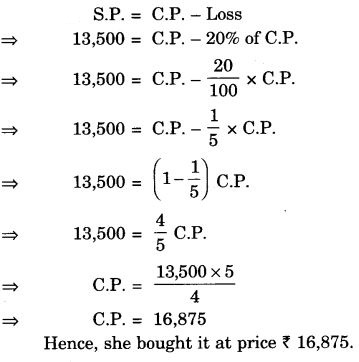Question 7.
(i) Chalk contains calcium, carbon, and oxygen in the ratio of 10 : 3 : 12. Find the percentage of carbon in chalk.
(ii) If in a stick of chalk, carbon is 3 g, what is the weight of the chalk stick?
Solution: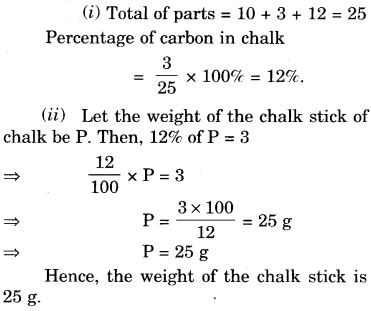Question 8.
Amina buys a book for ₹ 275 and sells it at a loss of 15%. How much does she sell it for?
Solution:
C.P. = ₹ 275
Loss = 15% of C.P = $$\frac { 15 }{ 100 }$$ × C.P
= $$\frac { 15 }{ 100 }$$ × 275
= ₹ 41.25
S.P. = C.P. – Loss = 275 – 41.25 = ₹ 233.75
hence, she se11 it for ₹ 233.75.

Question 9.
Find the amount to be paid at the end of 3 years in each case:

(a) Principal = ₹ 1,200 at 12% p.a.
(b) Principal = ₹ 7,500 aat 5% p.a.

Solution: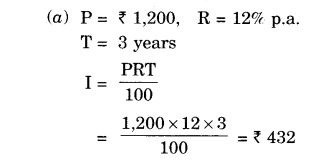Question 10.
What rate gives ₹ 280 as interest on a sum of ₹ 56,000 in 2 years?
Solution: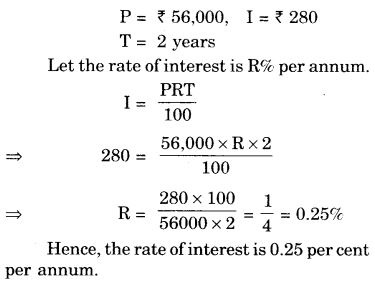Question 11.
If Meena gives an interest of ₹ 45 for one year at 9% rate p.a. What is the sum she has borrowed?
Solution:We hope the NCERT Solutions for Class 7 Maths Chapter 8 Comparing Quantities Ex 8.3 help you. If you have any query regarding NCERT Solutions for Class 7 Maths Chapter 8 Comparing Quantities Ex 8.3, drop a comment below and we will get back to you at the earliest.